Area Of Cylinder

Chapter 11 Class 9 Surface Areas and Volumes
Concept wise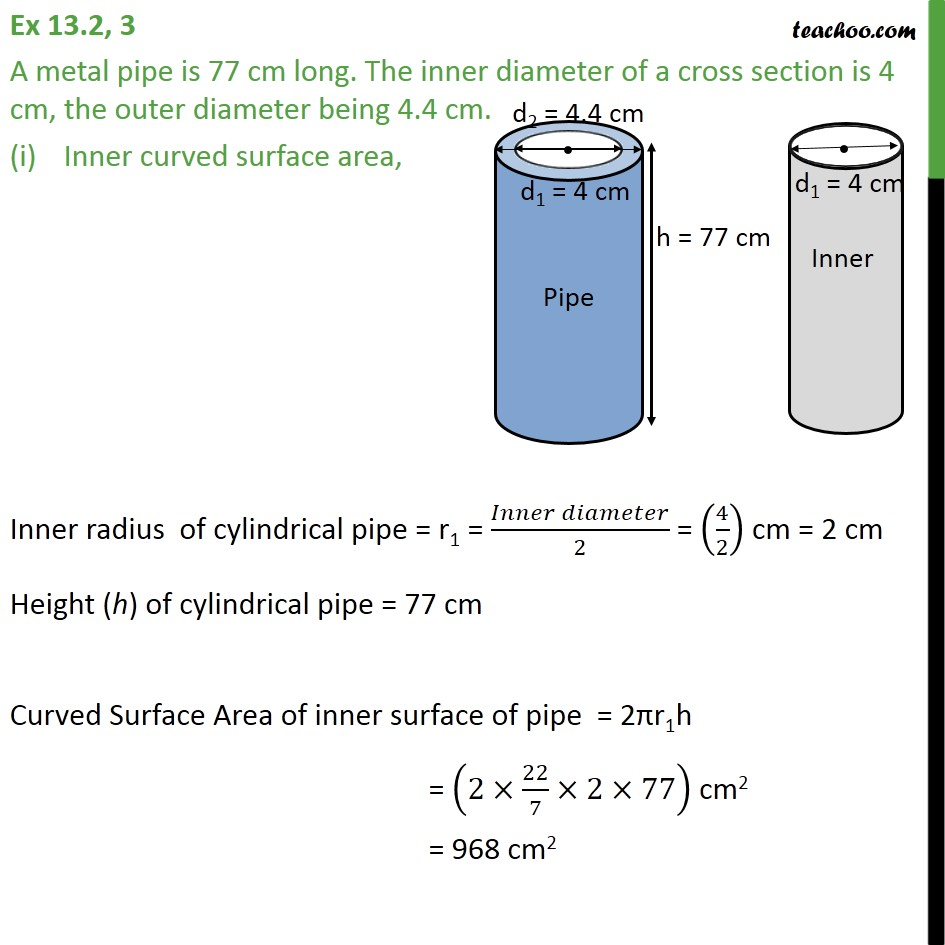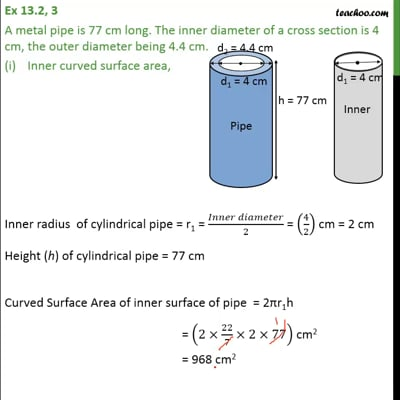This video is only available for Teachoo black users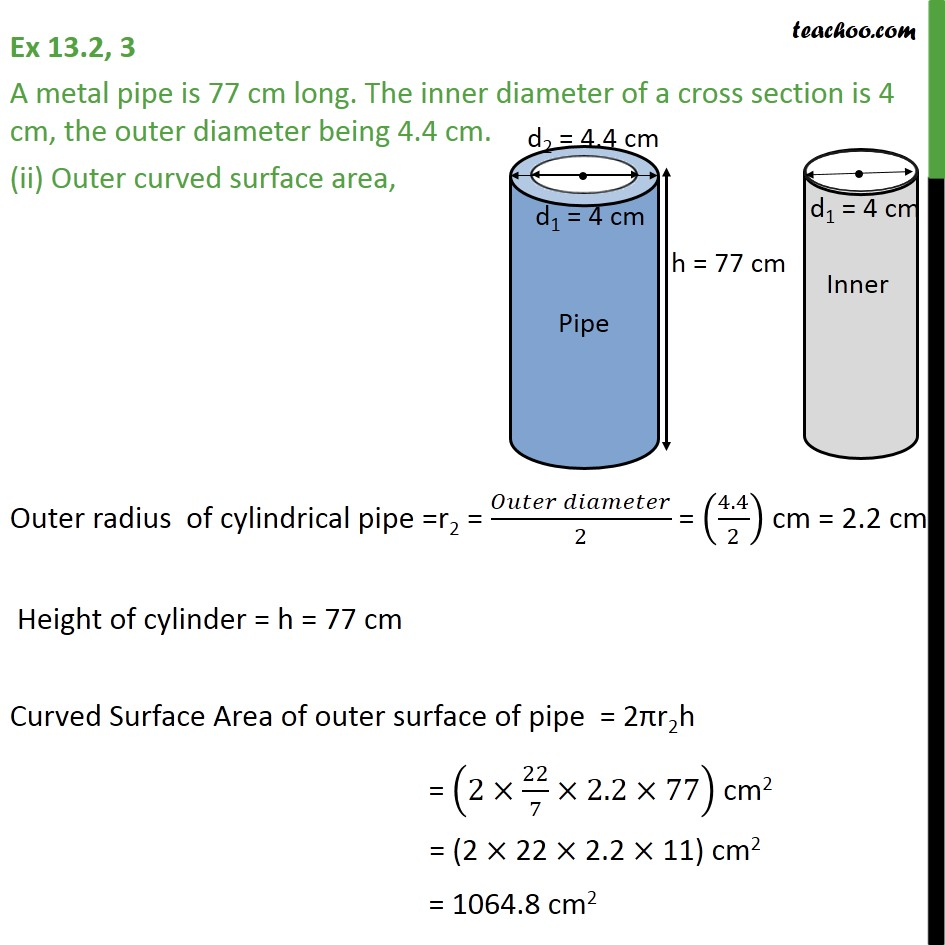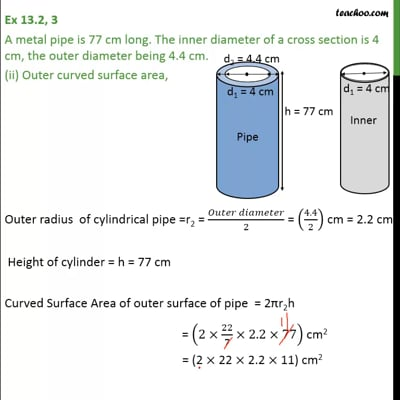This video is only available for Teachoo black users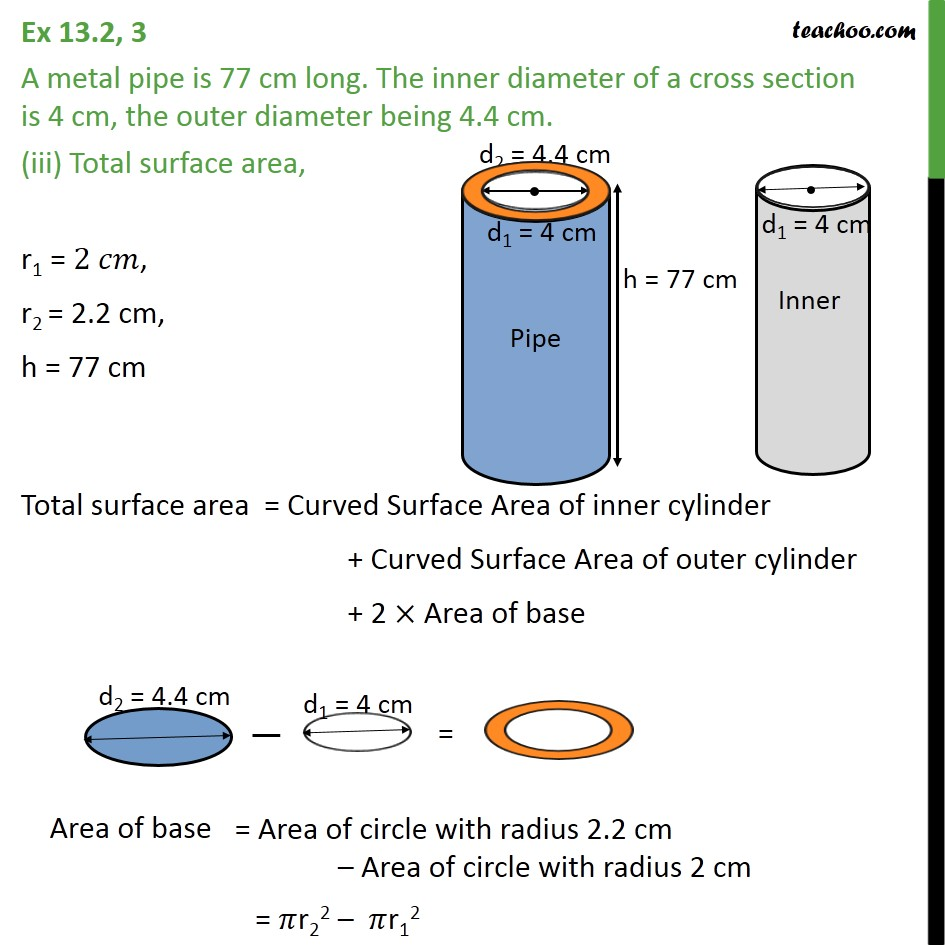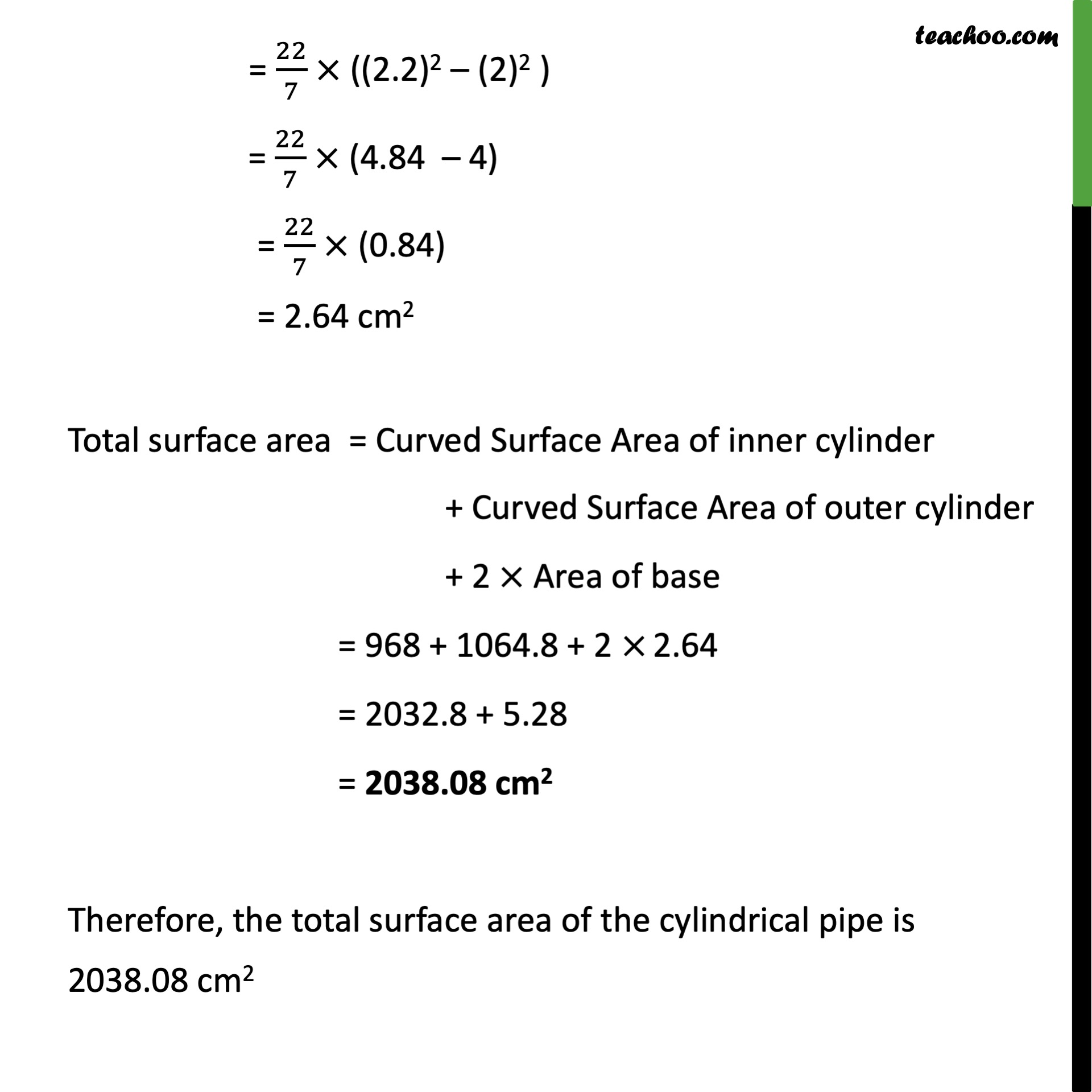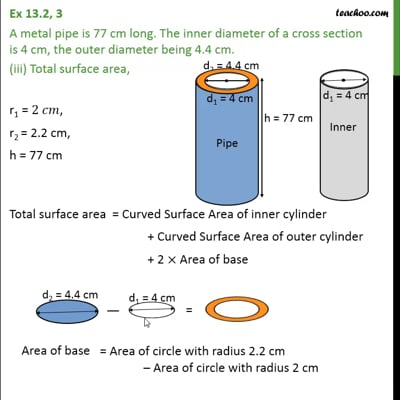This video is only available for Teachoo black users

Learn in your speed, with individual attention - Teachoo Maths 1-on-1 Class

### Transcript

Question 3 A metal pipe is 77 cm long. The inner diameter of a cross section is 4 cm, the outer diameter being 4.4 cm. Inner curved surface area, Inner radius of cylindrical pipe = r1 = ( )/2 = (4/2) cm = 2 cm Height (h) of cylindrical pipe = 77 cm Curved Surface Area of inner surface of pipe = 2 r1h = (2 22/7 2 77) cm2 = 968 cm2 Question 3 A metal pipe is 77 cm long. The inner diameter of a cross section is 4 cm, the outer diameter being 4.4 cm. (ii) Outer curved surface area, Outer radius of cylindrical pipe =r2 = ( )/2 = (4.4/2) cm = 2.2 cm Height of cylinder = h = 77 cm Curved Surface Area of outer surface of pipe = 2 r2h = (2 22/7 2.2 77) cm2 = (2 22 2.2 11) cm2 = 1064.8 cm2 Question 3 A metal pipe is 77 cm long. The inner diameter of a cross section is 4 cm, the outer diameter being 4.4 cm. (iii) Total surface area, r1 = 2 , r2 = 2.2 cm, h = 77 cm Total surface area = Curved Surface Area of inner cylinder + Curved Surface Area of outer cylinder + 2 Area of base Area of base = r22 r12 = 22/7 ((2.2)2 (2)2 ) = 22/7 (4.84 4) = 22/7 (0.84) = 2.74 cm2 Total surface area = Curved Surface Area of inner cylinder + Curved Surface Area of outer cylinder + 2 Area of base = 968 + 1064.8 + 2 2.74 = 2032.8 + 5.76 = 2038.08 cm2 Therefore, the total surface area of the cylindrical pipe is 2038.08 cm2